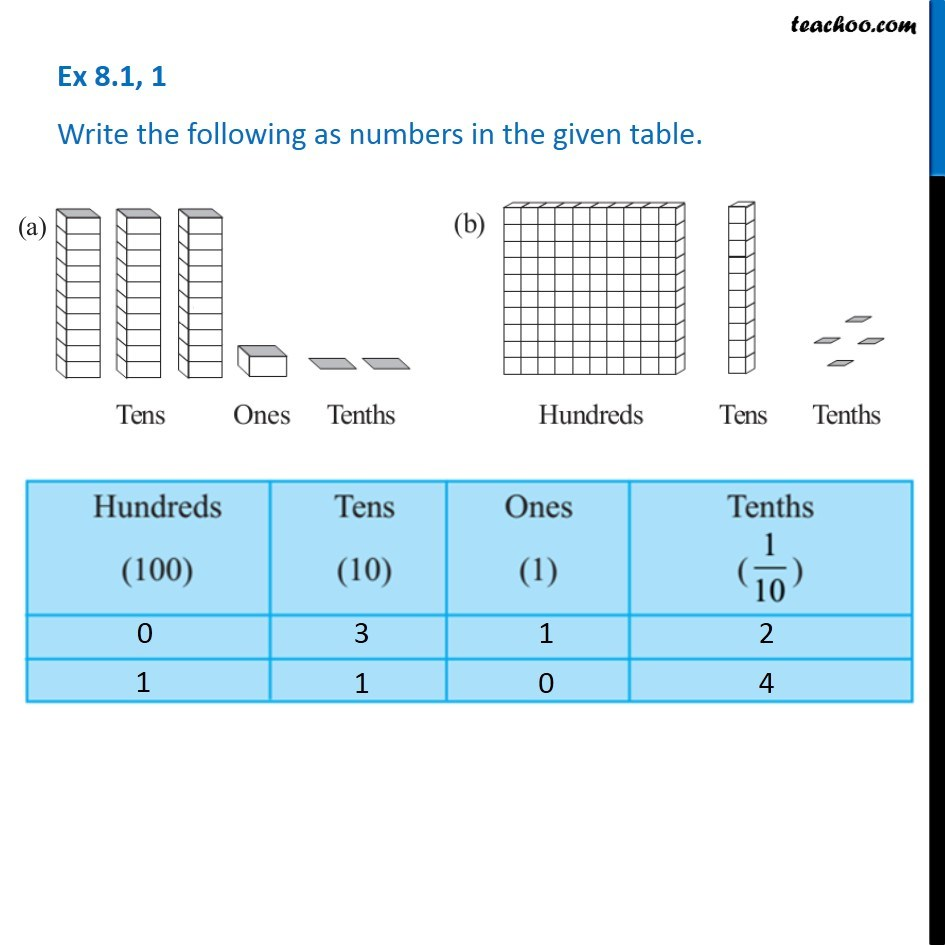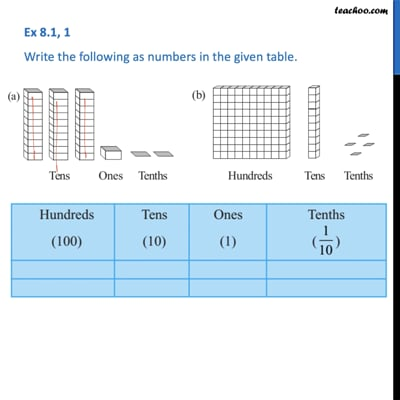Place Value Table

Chapter 8 Class 6 Decimals
Serial order wiseThis video is only available for Teachoo black users

Learn in your speed, with individual attention - Teachoo Maths 1-on-1 Class

### Transcript

Question 1 - Chapter 8 Class 6 Integers (NCERT Book) Write the following as numbers in the given table. For (a) Hundreds (100) = 0 Tens (10) = 3 Ones (1) = 1 Tenths (1/10) = 2 For (b) Hundreds (100) = 1 Tens (10) = 1 Ones (1) = 0 Tenths (1/10) = 4